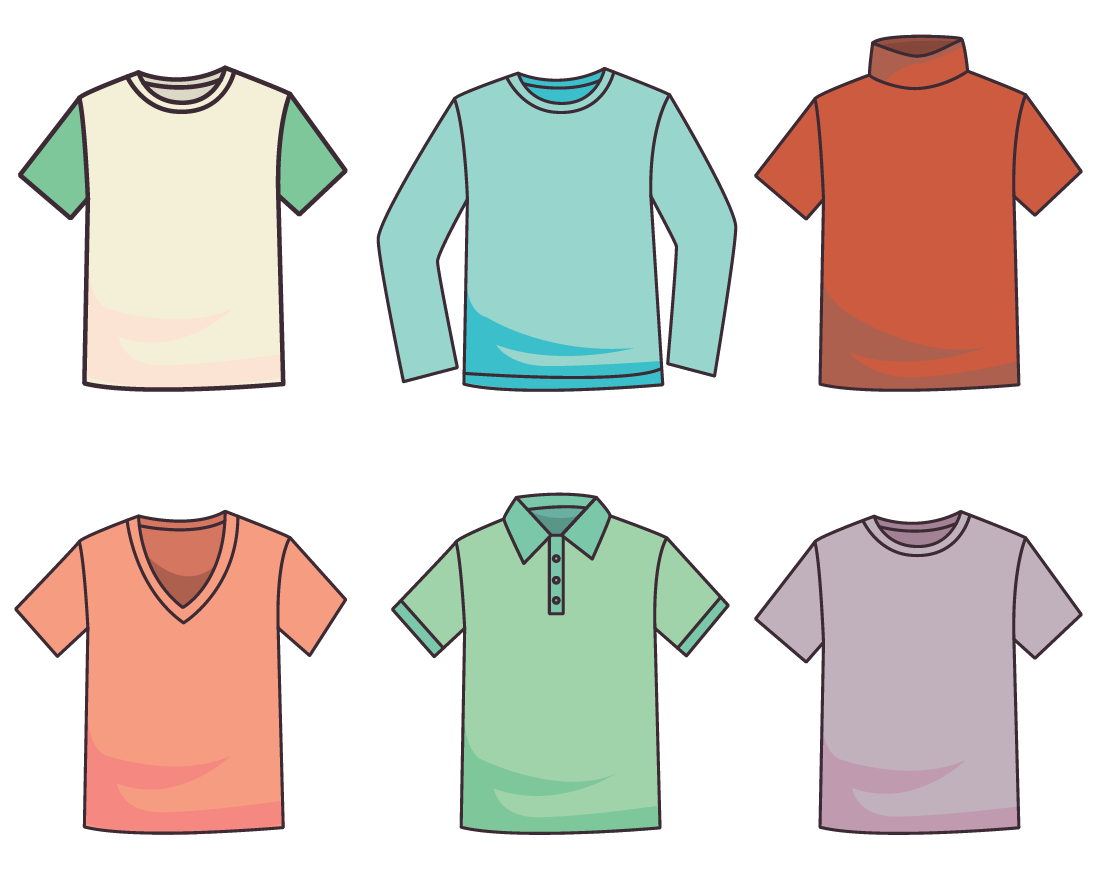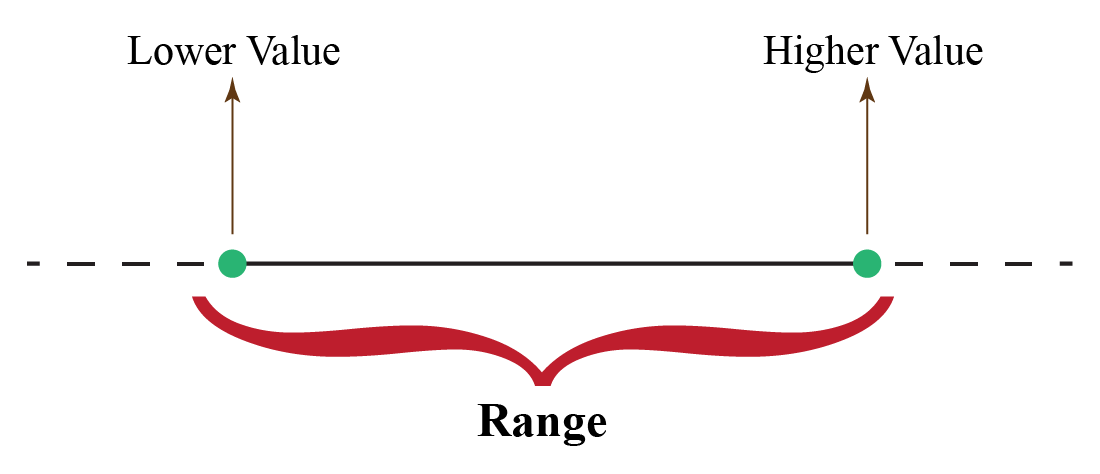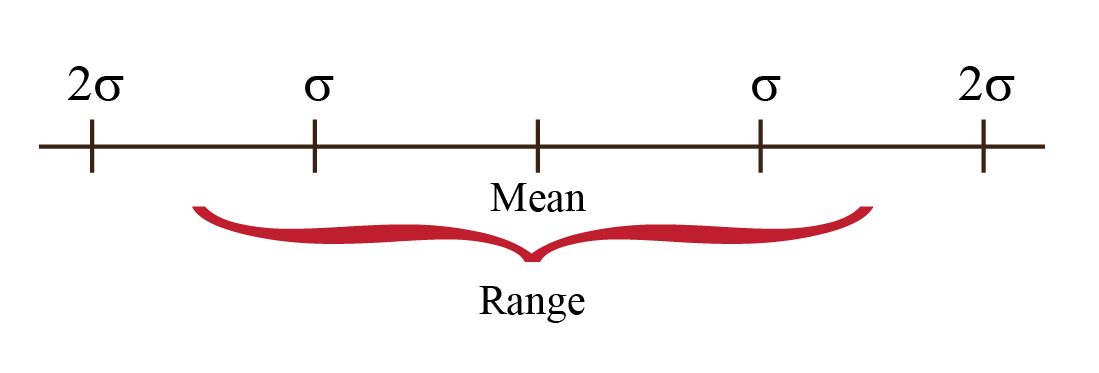# Range in Statistics

Range in Statistics

When you buy things, they are always sold within a price range. Take the example of your favourite pair of jeans. The store from where you made the purchase probably had a range of colors, a range of fits, a range of sizes, and a range of prices.

This range usually enables us to make a more informed decision about what exactly - in this case a pair of jeans - we want to buy.

Range is usually defined with an upper value and lower value and it refers to all the units between those values.With this example, let's move ahead to know more about range in statistics.

In this mini-lesson, we shall explore the topic of range in statistics, by finding answers to questions like what is meant by range in statistics, how to find range in statistics, what is the rule of thumb to find the range, and what are the limitations of range.

## Lesson Plan

 1 What is Meant by Range in Statistics? 2 Important Notes 3 Think out of the Box! 4 Solved Examples on Range in Statistics 5 Interactive Questions on Range in Statistics

## What is Meant by Range in Statistics?

The range is the difference between the highest value and the lowest value of the data.  It helps in knowing the spread of the data.$\text{Range = Highest Value - Lowest Value}$

### Example

Find the range of the data 2, 7, 11, 12, 19, 22, 25, 27, 33, 35

Highest Value = 35

Lowest Value = 2

Range = Highest Value - Lowest Value = 35 - 2 = 33

## What is Rule of Thumb?

As per the rule of thumb, the range of data lies within four standard deviations.  Two standard deviations above the mean and two standard deviations below the mean.

The formula for Standard Deviation $$(\sigma )$$ is as follows.  $\sigma = \sqrt{\dfrac{x_i - \mu}{N}}$## FAQs on Range in Statistics

### 1. What is the range in math?

The range in math is the difference between the highest value datapoint and the lowest value datapoint of the given data.

### 2. What is the use of range?

Range helps us to know the spread of the data. It also helps us to identify if the given data point is within the defined range or not.

### 3. What does the range in statistics tell us?

The range in statistics tells us the size of the data.  Also for a given end datapoint of the data, we can find the other end data point, with the help of range.

### 4. How do you find the range in statistics?

The process of finding range is statistics can be simplified in three quick steps.

1. Arrange the given data in ascending order or descending order.
2. Identify the lowest highest value of the data.
3. Find the difference between the lowest and the highest data point, to get the range.

### 5. Why is range important?

Range helps to define the spread of the data.  Knowing the range helps to define the scale to represent the data.

### 6. What is the practical utility of range?

The range is practically used to know the capacity.  Range helps to know the minimum and maximum capacity.

### 7. Do outliers count in range?

Outliers are generally included in the range.  Further to avoid outliers a new quantity called interquartile range is used.

More Important Topics
More Important Topics
Learn from the best math teachers and top your exams

• Live one on one classroom and doubt clearing
• Practice worksheets in and after class for conceptual clarity
• Personalized curriculum to keep up with school